# Connected sum of manifolds

(Redirected from Connected sum)

## Definition

Let$M_1$ and$M_2$ be connected manifolds. A connected sum of$M_1$ and$M_2$, denoted$M_1 \# M_2$, is constructed as follows. Let$f_i:\R^n \to U_i$ be homeomorphisms where$U_i$ are open subsets of$M_i$. Let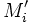$M_i'$ denote the complement in$M_i$ of the image of the open unit ball in$\R^n$, under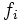$f_i$. Then the connected sum is the quotient of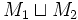$M_1 \sqcup M_2$ under the identification of the boundary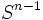$S^{n-1}$s with each other, via the composite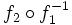$f_2 \circ f_1^{-1}$.

In general, the homotopy type of the connected sum of two manifolds depends on the choice of open neighbourhoods and on the way of gluing together. Further information: homotopy type of connected sum depends on choice of gluing map

## Homology

Further information: Homology of connected sum

The homology of the connected sum can be computed using the Mayer-Vietoris homology sequence for open sets obtained by enlarging the$M_i'$s slightly, and using the fact that$M_i'$ is a strong deformation retract of$M_i$ minus a point.

The interesting phenomena occur at$n$ and$n-1$, because this is where the gluing is occurring.